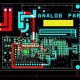# What is Power Amplifier & Its Types?

In this tutorial, we are going to learn about Power Amplifier & Its Types.

AmplifierAmplifier – An amplifier is an electronic device which through can increases voltage, current and power of a signal is called power amplifier.

Types of Amplifier:

1. Class A
2. Class B
3. Class AB
4. Class C

Class A – The class A amplifier is one in which the operating point and the input signal are such that the current in the output circuit flows at full times.

As per below image for amplifier of Class A, The output signal of class A amplifiers varies for a full 360o of the cycle.

Class B – The class B amplifier in one in which the operating point is at an extreme end of its characteristic, so that the quiescent power is very small. If the signal voltage is sinusoidal, amplification takes place for only one half a cycles.

As per diagram the class B circuit amplifier provides an output signal varying over one half the input signal cycle of for 180O if the input signal.

Class ABClass AB – The class AB amplifier which has one operating between the two extremes defined for class A and Class B. hence the output signal is zero for part but less than half of an input sinusoidal signal.

For class AB operating the output signal swing occurs between 180o and 360o and is neither class A nor class B operation.

Class C – A class C amplifier is one in which the operating point is chosen so that the output current or voltage is zero for more than one half of the input sinusoidal signal cycle.

The output of this amplifier is generally biased for operation at less than 180o of the cycle and will operate only with a tuned (resonant circuit) that’s provides a full cycle of operation for the tuned or resonant frequency.

Main Difference of all power class amplifiers: